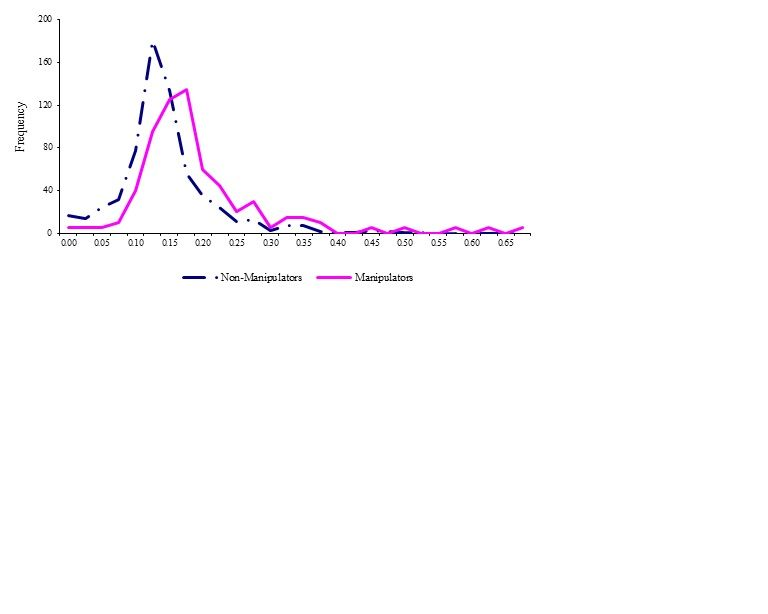## Graphing a frequency distributions chart to compare manipulator and non-manipulator

Hi,

I would like to graph a frequency distributions chart to compare the distribution of manipulator and non-manipulator.

However, the frequency of manipulator is really small, so i want to multiply a number on the frequency of distrubution for manipulator in order to allow easier visual comparison with the much larger sample of non-manipulator.

I use proc sgplot. How can I make it work?

Thank you very much for your help.

1 ACCEPTED SOLUTION

Accepted Solutions

## Re: Graphing a frequency distributions chart to compare manipulator and non-manipulator

If you want a kernel density estimate of the FREQ variable, you can use

``````proc sgplot data=em;
density freq / type=kernel group=em;
run;
``````

If you want to form a histogram, but plot the frequencies as a series, you can use PROC UNIVARIATE to bin the data and the SERIES statement to plot the binned frequencies:

``````proc univariate data=em noprint;
class em;
histogram freq  / outhist=out
midpoints=(0 to 0.8 by 0.05);
run;

proc sgplot data=out;
series x=_midpt_  y=_count_ / group=em;
run;``````
9 REPLIES 9

## Re: Graphing a frequency distributions chart to compare manipulator and non-manipulator

Instead of multiplying, plot both histograms or bar charts on the percentage scale. For example, this is how you can plot percentage of males and females on the same bar chart:

``````proc sgplot data=sashelp.class;
vbar age / group=sex stat=percent groupdisplay=cluster;
run;
``````

If you have a continuous variable, you can use  comparative histograms to compare the distribution across groups.

## Re: Graphing a frequency distributions chart to compare manipulator and non-manipulator

Thank you very much for your prompt response. But it has to be the frequency scale. D you know how to do it? thank you

## Re: Graphing a frequency distributions chart to compare manipulator and non-manipulator

I don't understand why you say "it has to be the frequency scale."  To compare absolute counts, plot the data on the count scale. To compare the relative proportions, plot on the percentage scale.

Do you have some sampling scheme in which you oversampled one category? For example, does each male in your data represent 10 members of the population but each female represents 100?  Even then, I think plotting proportions are the way to go.

## Re: Graphing a frequency distributions chart to compare manipulator and non-manipulator

Hi Rick,

Sorry for making you confuse.
I have matched one manipulator to five non-manipulator, and i need to graph a frequency distribution char to compare the difference of ditribution between manipulator and non-manipulator.

Please find the attached figure. I need to do the same thing as the enclosed figure.

Thank you very much for your help.## Re: Graphing a frequency distributions chart to compare manipulator and non-manipulator

Well, I still don't like it, but you can do it by using a FREQ variable. Here is an example in which each female in the data set  represents 5 females:

``````data FakeData;
set sashelp.class;
if sex="F" then freq=5;
else freq=1;
run;

proc sgplot data=FakeData;
vbar age / group=sex freq=freq groupdisplay=cluster;
run;``````

## Re: Graphing a frequency distributions chart to compare manipulator and non-manipulator

Thank you for your help, though it is still different from what i need.

Regards,

## Re: Graphing a frequency distributions chart to compare manipulator and non-manipulator

If you want a line instead of bars, just use the VLINE statement instead of VBAR.

If you have a continuous variable, use the DENSITY statement.

If you post some sample data, I'm sure that someone can give you what you need.

## Re: Graphing a frequency distributions chart to compare manipulator and non-manipulator

Thank you. the following is the sample data. i want to compare the difference of  distribution (frequency) of Estimated probability between em=1 and em=0.

 Global Company Data Year - em Estimated Probability Key Fiscal 1173 1999 1 0.50883 137377 1999 0 0.53093 1173 1999 1 0.50883 31015 1999 0 0.36718 1173 1999 1 0.50883 8388 1999 0 0.45892 1173 1999 1 0.50883 133729 1999 0 0.52904 1173 1999 1 0.50883 61553 1999 0 0.6904 1173 2000 1 0.48688 63038 2000 0 0.53392 1173 2000 1 0.48688 26038 2000 0 0.67335 1173 2000 1 0.48688 15758 2000 0 0.56561 1173 2000 1 0.48688 62874 2000 0 0.49828 1173 2000 1 0.48688 143526 2000 0 0.48667 1173 2001 1 0.48896 15758 2001 0 0.43567 1173 2001 1 0.48896 2299 2001 0 0.4265 1173 2001 1 0.48896 24982 2001 0 0.52106 1173 2001 1 0.48896

## Re: Graphing a frequency distributions chart to compare manipulator and non-manipulator

If you want a kernel density estimate of the FREQ variable, you can use

``````proc sgplot data=em;
density freq / type=kernel group=em;
run;
``````

If you want to form a histogram, but plot the frequencies as a series, you can use PROC UNIVARIATE to bin the data and the SERIES statement to plot the binned frequencies:

``````proc univariate data=em noprint;
class em;
histogram freq  / outhist=out
midpoints=(0 to 0.8 by 0.05);
run;

proc sgplot data=out;
series x=_midpt_  y=_count_ / group=em;
run;``````
Discussion stats
• 9 replies
• 7757 views
• 0 likes
• 2 in conversation